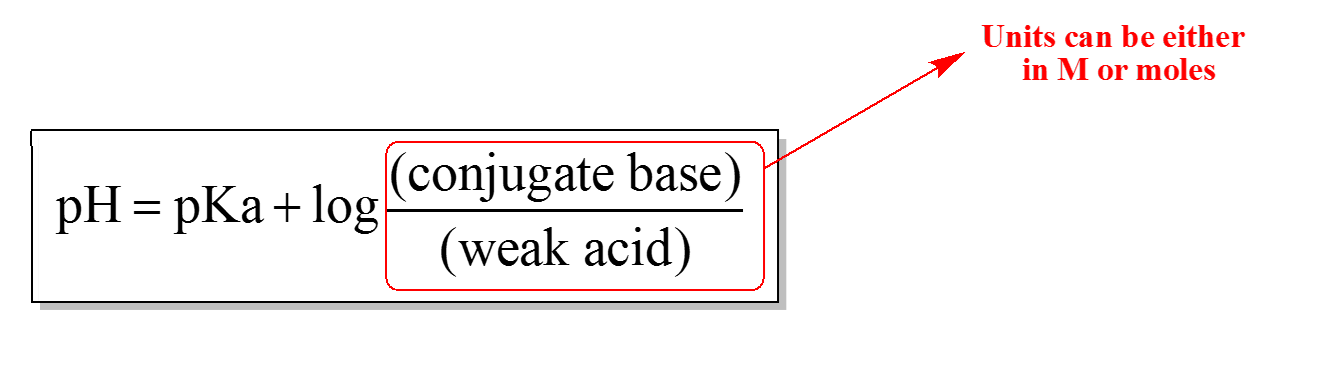Clutch Prep is now a part of Pearson
Ch.10 Acids and BasesWorksheetSee all chapters

# Henderson-Hasselbalch Equation

See all sections
Sections
Acid-Base Introduction
Arrhenius Acid and Base
Bronsted Lowry Acid and Base
Acid and Base Strength
Ka and Kb
The pH Scale
Auto-Ionization
pH of Strong Acids & Bases
Acid-Base Equivalents
Acid-Base Reactions
Gas Evolution Equations (Simplified)
Ionic Salts (Simplified)
Buffers
Henderson-Hasselbalch Equation
Strong Acid Strong Base Titrations (Simplified)

Concept #1: The Henderson – Hasselbalch Equation.

Transcript

Hey guys, in this new video, we’re finally get to see how do we calculate the pH of a buffer. We’re going to say we learned that whenever we had a weak acid or a base, we have to use our favorite friend, the ICE chart in order to find pH or pOH. The great thing is now that we're dealing with buffers, we no longer necessarily need to do an ICE chart. We're going to say anytime we know we have a buffer for sure, we can skip the Ice chart altogether and just use our Henderson – Hasselbalch equation. So, Henderson-Hasselbalch. It's also known as the buffer equation. It says that pH equals pKa plus the log of conjugate base over weak acid. When I say pKa, remember P just means negative log. pKa equals the negative log of Ka. Here when I’m talking about conjugate base or weak acid, the units here can be either in molarity or in moles. It all depends. If they give you only molarity, use molarity. If they give you volumes of a particular molarity, remember the word of means multiply. We’d multiply liters times molarity to give ourselves moles.Example #1: What is the pH of a solution consisting of 2.75 M sodium phenolate (C6H5ONa) and 3.0 M phenol (C6H5OH). The Ka of phenol is 1.0 x 10-10.

Transcript

Let's look at this first question and see how exactly do we use it. Here it says: What is the pH of a solution consisting of 2.75 molar sodium phenolate, which is this compound, and 3.0 molar phenol, which is this compound. The Ka of phenol is 1.0 times 10 to the negative 10. What you should realize here is phenol has what? It has H's, non-metals, and oxygen. Phenol is an oxyacid. Use the Math that we've learned. You take the number of oxygens minus by the number of hydrogens. We don't get two oxygens left, so this is a weak oxyacid. We definitely know it’s weak because look at its Ka. Its Ka value is extremely small. If your Ka value is less than 1, you're a very weak acid.
Here we have a weak acid. sodium phenolate has one less H available, so this is the conjugate base. We have a weak acid, we have a conjugate base and therefore, we have a buffer. Because we have a buffer, we need to use the buffer equation, pH equals negative log of Ka plus the log of, now they only gave us the molarity here, so that's what we're going to plug in: 2.75 molar the conjugate base over 3.0 molar, our weak acid. When we plug all that in, we’ll get back the answer of 9.96

Practice: Calculate the pH of a solution formed by mixing 200 mL of a 0.400 M C2H5NH2 solution with 350 mL of a 0.450 M C2H5NH3solution. (Kb of C2H5NHis 5.6 x 10 -4).

Example #2: What is the buffer component concentration ratio, [Pr ] / [HPr] , of a buffer that has a pH of 5.11. (The Ka of HPr is 1.30 x 10-5).

Example #3: Over what pH range will an oxalic acid (H2C2O4) / sodium oxalate (NaHC2O4) solution work most effectively? The acid dissociation constant of oxalic acid is 6.0 x 10-2.

a) 0.22 – 2.22        b) 1.00 – 3.00        c) 0.22 – 1.22        d) 2.0 – 4.0

Practice: Determine how many grams of sodium acetate, NaCH3CO2 (MW: 82.05 g/mol), you would mix into enough 0.065 M acetic acid CH3CO2H (MW: 60.05 g/mol) to prepare 3.2 L of a buffer with a pH of 4.58. The Ka is 1.8 x 10-5.

Example #4: Which weak acid-conjugate base combination would be ideal to form a buffer with a pH of 4.74.

a)     Cyanic acid and Potassium cynate           (Ka = 4.9 x 10-10)

b)     Benzoic acid and Lithium benzoate         (Ka = 6.3 x 10-5)

c)     Acetic acid and Sodium acetate               (Ka = 1.7 x 10-5)

d)     Ammonium chloride and Ammonia         (Ka = 5.56 x 10-10)

e)     Formic acid and Cesium formate             (Ka = 1.7 x 10-4)

Practice: A buffer solution is made by combining a weak acid with its conjugate salt. What will happen to the pH if the solution is diluted to one-fourth of its original concentration?